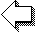## FORTRAN Transcompiler

In 1957, IBM released the first FORTRAN compiler for the IBM 704 mainframe computer. The early language had very limited features: simple I/O, arithmetic IF conditionals, DO loops, and GOTO statements. The only supported variable types were integer, real, and arrays.

As the first language version of FORTRAN is more than 60 years old, no compiler for modern platforms exists. Fortunately, a source-to-source compiler has been written in Fortran 2008 that translates FORTRAN code to ANSI C. The C code can then be compiled to native binaries.

### Build the Compiler

At first, download the source code of the transcompiler from GitHub:

``\$ git clone https://github.com/interkosmos/fif.git``

Just execute BSD make to build it:

``\$ make``

Then, compile your FORTRAN I code by running:

``\$ ./compiler <input> <output>``

### Compile FORTRAN code

Let us take a simple example written in FORTRAN I and compile it with the transcompiler. The program just calculates the average of entered values:

``````C     AVERAGE.F
C     PROGRAM FOR CALCULATING THE AVERAGE VALUE
C    X    OF A SET OF NUMBERS
DIMENSION A(999)
1 FORMAT (I3/(F6.3))
READ 1 N, (A(J), J = 1, N)
SUM = 0.0
DO 6 I = 1, N
IF (A(I)) 5, 2, 2
2 SUM = SUM + A(I)
3 FORMAT (F6.3)
PRINT 3 A(I)
CONTINUE
4 FORMAT (21HNEGATIVE NUMBER FOUND)
5 PRINT 4
6 CONTINUE
7 FORMAT (9HAVERAGE: F6.3)
PRINT 7 SUM / N``````

Save the source code as `average.f`. Compile and run the program with:

``````\$ ./compiler average.f average
\$ ./average``````

At first, the user has to input the number of values to average, and hit return. Then, the floating-point numbers do follow one by one, separated by space. Here, the average of five numbers has been calculated:

``````\$ ./average
5
21.4 43.3 12.9 6.4 41.2
21.400
43.300
12.900
6.400
41.200
AVERAGE:  25.040``````

Please see the Programmer’s Reference Manual for more examples.

### References

Last Update: Sat, 03 Oct 2020 11:55:10 +0200